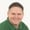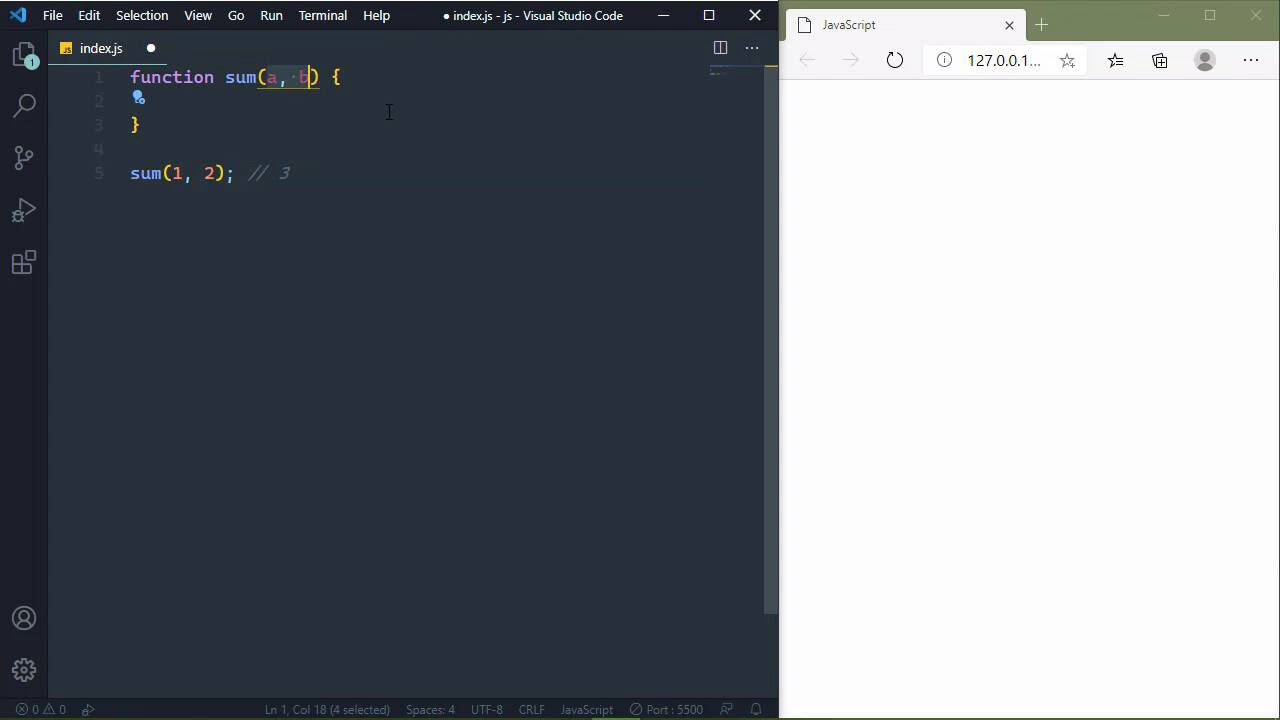7 days of WordPress plugins, themes & templates - for free!* Unlimited asset downloads! Start 7-Day Free TrialFREELessons: 33Length: 4.8 hours• Overview
• Transcript

# 5.2 Writing Your Own Functions

Every page or application we write will more than likely require our own functionality. So you'll need to write your own functions, and you'll learn how in this lesson.

## 11.Conclusion1 lesson, 01:47

### 5.2 Writing Your Own Functions

In the previous lesson, we very briefly looked at functions and variables. And that they are two very important parts of not just JavaScript, but any programming language. But when it comes to running our own software, well, we're going to be writing our own functions. I mean the built-in functions that are given to us by JavaScript and through the browser are useful. I mean, alert does serve some purpose, however, we want our own functionality. For example, let's say that we have been assigned a project to where we have to write a calculator. Well, the alert function isn't going to really help us there and really nothing that comes from JavaScript or comes from the browser is going to help us. So we need to write our own functionality. Because if you think about it, we have at least four functions that we would need to write. We need to add, subtract, multiply, and divide. Now, we're not going to implement all those but lets write a function for adding two numbers together. So to create a function, kind of like a variable where we started with let. Well, we have a special word called function, and this basically creates a function anytime that we use it. So we have function followed by the name of the function and then a pair of parentheses. And then we want the code that is going to execute whenever we call the function and that is inside of these curly braces. Now, if you're brand new to programming, yeah, this is rather cryptic, this is a C style programming syntax. But of course as with everything, the more that you practice and use it, the more it becomes second nature to you. So right now, we have just defined our own function. And if we wanted to, we could call it just like we called the alert function in the previous lesson. We just need the name of our function and then we followed that up with a pair of parentheses. Now, it's not going to do anything because the function doesn't do anything, there's no code inside of it. And there is no data that is being input into that function. Kinda like the alert function, we had to give it a message in order for it to do its job. Well, we have to do the same thing for the sum function, we need to pass in the two numbers that we want to add together. So whenever we define a function, we can also define the input that is going to be used by that function. So we have two numbers, 1 and 2. And we could create what we call parameters called a and b to represent those numbers. These are essentially variables that represent the numbers that are passed to our sum function. And this gives us the ability to work with those values. So that's inside of our sum function, we can take a + b, and there we go, we've added them together. So if we wanted to, we could call alert here and we could pass in a + b, and whenever we save this on the right hand side, we will see the value of of 3. However, most of the time we want to call a function and we want it to return a value so that we can then work with it. For example, let's say that we want to create a variable called three and we want to assign that variable the result of calling our sum function. Well, that's very easy to do because we can return a value from inside of a function. So in this case, we can return a + b that is going to take a plus b, add them together, and then return that value to whatever we are assigning it to. So if we want it to alert the value of the three variable, we will see three. And of course, that's going to change based upon the values that we pass to our sum function. So if we pass 5 and 2, well, we're going to see 7 there. But let's do this, let's create another variable called seven. And we will call the sum function, we will pass in 5 and 2, that will give us the value of seven so that we can then display that. So the beauty about a function is that we can call it as many times as we want. In fact, that's the purpose of why we would want to write a function so that we could reuse that functionality over and over again. And so if we wanted to write a subtract function, we would do the same thing function subtract, then we would have our parentheses. But let's go ahead and define what we call parameters, the input that our function is going to use. And we will return a- b. And then, if we wanted to use that, let's just call that one, and we will subtract 3 and 2. Let's display the result of that, and that will, of course, be 1. Well, in the next lesson, we are going to talk about more complex things. We've talked about variables, which is just an individual's storage for a value. We've talked about functions which are just a single thing that performs work. Well, an object combines all of that together, and you will see how in the next lesson

Back to the top